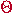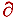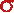Math & Science Home | Proficiency Tests | Mathematical Thinking in Physics | Aeronauts 2000

 CONTENTS Introduction Fermi's Piano Tuner Problem How Old is Old? If the Terrestrial Poles were to Melt... Sunlight Exerts Pressure Falling Eastward What if an Asteroid Hit the Earth Using a Jeep to Estimate the Energy in Gasoline How do Police Radars really work? How "Fast" is the Speed of Light? How Long is a Light Year? How Big is a Trillion? "Seeing" the Earth, Moon, and Sun to Scale Of Stars and Drops of Water If I Were to Build a Model of the Cosmos... A Number Trick Designing a High Altitude Balloon Pressure in the Vicinity of a Lunar Astronaut Space Suit due to Outgassing of Coolant Water Calendar Calculations Telling Time by the Stars - Sidereal Time Fields, an Heuristic Approach The Irrationality ofThe Irrationality ofThe Number (i)i Estimating the Temperature of a Flat Plate in Low Earth Orbit Proving that (p)1/n is Irrational when p is a Prime and n>1 The Transcendentality ofIdeal Gases under Constant Volume, Constant Pressure, Constant Temperature and Adiabatic Conditions Maxwell's Equations: The Vector and Scalar Potentials A Possible Scalar Term Describing Energy Density in the Gravitational Field A Proposed Relativistic, Thermodynamic Four-Vector Motivational Argument for the Expression-eix=cosx+isinx Another Motivational Argument for the Expression-eix=cosx+isinx Calculating the Energy from Sunlight over a 12 hour period Calculating the Energy from Sunlight over actual full day Perfect Numbers-A Case Study Gravitation Inside a Uniform Hollow Sphere Further note on Gravitation Inside a Uniform Hollow Sphere Pythagorean Triples Black Holes and Point Set Topology Additional Notes on Black Holes and Point Set Topology Field Equations and Equations of Motion (General Relativity) The observer in modern physics A Note on the Centrifugal and Coriolis Accelerations as Pseudo Accelerations - PDF File On Expansion of the Universe - PDF File

##### Calculating the Energy from Sunlight over a 12-Hour Period

(Written in response to an inquiry recently received)

Incident sunlight is usually thought of in terms of power per unit area. The typical units are mW/cm2. At the earth's surface, the nominal value of the solar constant is 137 mW/cm2. This value corresponds to high noon with the sun directly overhead (as would occur at the equator or in the tropics).

The energy from sunlight may be obtained from this number and a little geometry. If we take energy in mJ (millijoules), then from the units alone we obtain

mJ = (mW/cm2) x (Area in cm2) x (Time in sec)

(since mW = mJ/sec). If the sun were always directly overhead, the amount of energy incident upon a 1 cm2 solar collector oriented perpendicular to the sun's rays in 12 hours would be

(137 mW/cm2) x (1 cm2) x (4.3 x 104 sec.) = 5.9 x 106 mJ.

But we know that the sun is usually not directly overhead. It moves East-West throughout the course of a day and North-South throughout the course of a year. The yearly variation is easily taken care of. We know that the sun moves ± 23.5° above and below the equator over the course of a year. Typically, it is on the equator every March 21 and September 21. We may estimate, the sun's position north of theequator, by calculating= 23.5° sin (2{T/365.25})

where dT is the number of days counted from the vernal equinox (April 21). (Notice that for northern winter months,is negative). We may them estimate the solar constant for any particular day by calculatingD = (137mW/cm2) cos (L -)

where L is our latitude.

The daily variation is a little more involved. Let the sun be at altitude A (angle above the eastern horizon) at a given time t during the day. Then at time t + dt, the altitude will have increased to A + dA. The differential time is related to the differential altitude by

dt = (12/) dA hr

since the sun travels throughradians (180°) in 12 hours.
If we again try to calculate the amount of energy incident upon a 1 cm2 solar collector oriented perpendicular to the sun's noon-time rays in 12 hours, we would have to use for the equivalent collecting area
Area = (sin A) cm2
since we want only the amount of area projected perpendicular to the sun's rays at time t. The differential energy dE incident upon our solar collector in time dt is

dE =D (sin A) dt
=D (sin A) (12/) (3,600 sec/hr) dA
=D (4.3 x 104 sec/) [(sin A) dA].

The expression in square brackets may be integrated from A = 0 to A =to yield

E =D (4.3 x 104 sec/)

If, for comparison, we setD = 137 mW/cm2 (i.e., we were to take our collector to the tropics), we find

E = 3.7 x 106 mJ.

The ratio between this value and the one derived above is 2/. At my latitude, 42° N, the midsummer value is

E = 1.8 x 106 mJ

and the midwinter value is

E = 7.2 x 105 mJ.

The ratio of the midwinter to the midsummer values is

(7.2 x 105 mJ)/( 1.8 x 106 mJ) = 0.4,

which means that over a 12-hour period my solar collector collects a mere 40% of the energy in midwinter that it would in midsummer. And this particular calculation still does not take into account the longer days in summer and the shorter days in winter! The next article will tell how that calculation is to be done...